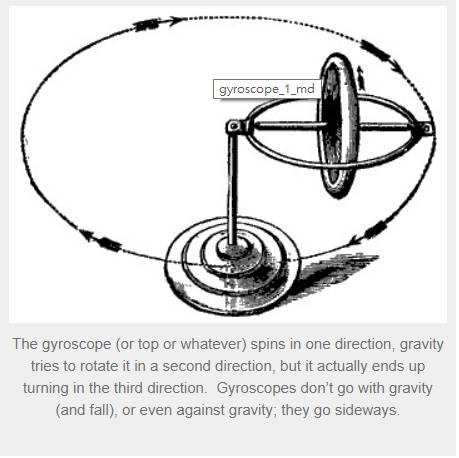# Simple derivation of the Equations of a Gyroscope

• B
alan123hk
Frankly, it is difficult for me to understand the derivation of mathematical equations that describe and explain the motion behavior of a gyroscope, particularly the reason why it spins in one direction, gravity tries to rotate it in a second direction, but it actually ends up turning in the third direction and dose not fall down.

I'm looking for an as simple and easy to understand as possible way for deriving the equations of gyroscope to help me better understanding the basic principle of it.

Any help would be appreciated.#### Attachments

Homework Helper
Gold Member
2022 Award
Frankly, it is difficult for me to understand the derivation of mathematical equations that describe and explain the motion behavior of a gyroscope, particularly the reason why it spins in one direction, gravity tries to rotate it in a second direction, but it actually ends up turning in the third direction and dose not fall down.

I'm looking for an as simple and easy to understand as possible way for deriving the equations of gyroscope to help me better understanding the basic principle of it.

Any help would be appreciated.
View attachment 236714

That's an interesting way to look at it. The gyroscope is supported on its stand. If there were no stand it would fall down! The vertical forces are balanced: gravity down from the centre of mass and the normal force up through the stand. This, however, creates a "torque", which you would normally expect to rotate the object about the point of contact on the stand. Torque creates a change in angular momentum. As the gyroscope is spinning you have to take the change in that spin angular momentum into account, when you see that the torque is in the wrong direction to rotate a spinning gyroscope down.

The gyroscope is an example of the full vector nature of angular momentum. And that fact that you cannot ignore spin angular momentum in a rigid body.

•nnunn and alan123hk
I'm looking for an as simple and easy to understand as possible way for deriving the equations of gyroscope to help me better understanding the basic principle of it.

If linear momentum is more intuitive than angular momentum to you, it might help to decompose the gyroscope into point masses, and consider their linear momentum:

The angular momentum explanation mentioned by @PeroK is a bit more abstract:
http://hyperphysics.phy-astr.gsu.edu/hbase/rotv2.html

•PeroK
Homework Helper
Gold Member
2022 Award
If linear momentum is more intuitive than angular momentum to you, it might help to decompose the gyroscope into point masses, and consider their linear momentum:

The angular momentum explanation mentioned by @PeroK is a bit more abstract:
http://hyperphysics.phy-astr.gsu.edu/hbase/rotv2.html

There's a critical point at 2:25 where he equates the torque generated by the vertical forces to a torque generated by horizontal forces. That equivalence would appear to require that the wheel is supported by a rigid joint with the rope. In which case that equivalence would apply to a non-spinning wheel as well. Why can he equate the different torques for a spinning wheel but not for a non-spinning wheel? That would be my question.

Edit: to answer my own question, it doesn't require the rigid joint. In the case of the static wheel, the wheel rotates and falls; and, in the case of the spinning wheel, the wheek precesses. And the faster the wheel is spinning the more pronounced the precession and the less pronounced the falling.

Last edited:
alan123hk
Hi Perok, AT

Thanks for your valuable and informative replies.

I just found some very cool videos which explained the strange behavior of gyroscopes, and would like to share them to those who want to understand the basic physics of gyroscope. It should be useful for layman like me but certainly not for expert.
I think these videos are very cool because they clearly explained the principle of gyroscope in detail by means of simple and beautiful maths.

Last edited:
•nnunn